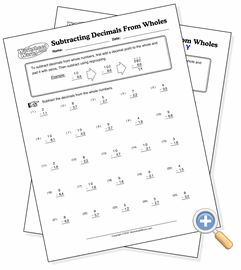# Decimals From Whole Numbers

## Decimal SubtractionUse regrouping to subtract decimal numbers from whole numbers

These problems provide practice in subtracting decimal numbers from whole numbers. The whole numbers will need to be padded with zeros and then regrouping used to perform the subtraction.